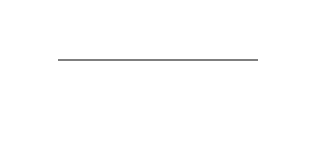Related Articles

# Which methods are used to draw a straight line on a Canvas ?

• Last Updated : 10 Aug, 2021

HTML provides a very interesting element known as <canvas>, it is only a container that is used to draw graphics on web pages, rest of the work is done with JavaScript.

There are several methods in JavaScript to draw shapes on the canvas. In this article, we will see how we can draw a line on a canvas and the essential methods used to draw a straight line.

There are the following methods to draw a straight line on the canvas.

1. beginPath(): This method is used to begin the path that we are going to draw. It does not take any arguments.

2. moveTo(): This method takes two arguments which will be the starting point of any path. The method will start the shape from that particular point.  We can later change the starting point by changing the values of its arguments.

Example:

` moveTo(10 , 30);`
3. lineTo(): This method will also take two values and it will add the starting point to the points that it holds. The code represents a path from one point to another point.

Example:

```moveTo(10 , 30);
lineTo(40 , 100);```
4. stroke(): This method will stroke the given path and make it visible, this method does not take any arguments.

All the methods that are listed above will be required to draw a line on the canvas. Note that these are only some methods that will be used to draw lines but there are several other methods available in JavaScript to work with canvas.

Example: Now create an HTML file and add the following code to draw a straight line on the canvas.

## HTML

 ```<``html` `lang``=``"en"``>`` ` `<``head``>``    ``<``meta` `charset``=``"UTF-8"``>``    ``<``meta` `http-equiv``=``"X-UA-Compatible"` `content``=``"IE=edge"``>``    ``<``meta` `name``=``"viewport"` `content``=``        ``"width=device-width, initial-scale=1.0"``>```` ` `<``body``>`` ` `    ````    ``<``canvas` `id``=``"canvas1"` `width``=``"500"` `height``=``"500"``>`` ` `    ``<``script``>``        ``var canvas = document.getElementById('canvas1');`` ` `        ``// Use the if condition in case any browser``        ``// does not support canvas``        ``if (canvas.getContext) {``            ``var context = canvas.getContext('2d');`` ` `            ``// Begin the path``            ``context.beginPath();`` ` `            ``// Starting point``            ``context.moveTo(5, 52);`` ` `            ``// End point``            ``context.lineTo(325, 55);`` ` `            ``// Stroke will make the line visible``            ``context.stroke();``        ``}``    `````` ` ``

Output:output

When working with canvas we have to give the size so that we can draw shapes inside it. Note that if the values of shapes excess the canvas boundary then the shape will be limited to that border and can’t go through, although it won’t give any error. So this is how we can draw lines on the canvas.

Attention reader! Don’t stop learning now. Get hold of all the important HTML concepts with the Web Design for Beginners | HTML  course.

My Personal Notes arrow_drop_up